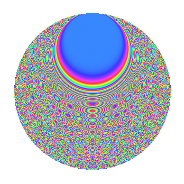# Properties

 Label 287.2.beLevel 287 Weight 2 Character orbit be Rep. character $$\chi_{287}(12,\cdot)$$ Character field $$\Q(\zeta_{120})$$ Dimension 832 Newforms 1 Sturm bound 56 Trace bound 0

# Related objects

## Defining parameters

 Level: $$N$$ = $$287 = 7 \cdot 41$$ Weight: $$k$$ = $$2$$ Character orbit: $$[\chi]$$ = 287.be (of order $$120$$ and degree $$32$$) Character conductor: $$\operatorname{cond}(\chi)$$ = $$287$$ Character field: $$\Q(\zeta_{120})$$ Newforms: $$1$$ Sturm bound: $$56$$ Trace bound: $$0$$

## Dimensions

The following table gives the dimensions of various subspaces of $$M_{2}(287, [\chi])$$.

Total New Old
Modular forms 960 960 0
Cusp forms 832 832 0
Eisenstein series 128 128 0

## Trace form

 $$832q - 16q^{2} - 48q^{3} - 20q^{4} - 48q^{5} - 32q^{7} - 48q^{8} - 24q^{9} + O(q^{10})$$ $$832q - 16q^{2} - 48q^{3} - 20q^{4} - 48q^{5} - 32q^{7} - 48q^{8} - 24q^{9} - 36q^{10} - 16q^{11} - 48q^{12} - 36q^{14} - 88q^{15} - 92q^{16} - 84q^{17} - 12q^{18} - 72q^{19} + 8q^{21} + 16q^{22} - 20q^{23} - 20q^{25} - 24q^{26} - 16q^{28} - 96q^{29} + 56q^{30} - 60q^{31} - 68q^{32} - 108q^{33} - 32q^{35} + 24q^{37} - 132q^{38} - 16q^{39} - 16q^{43} + 112q^{44} - 60q^{45} + 24q^{46} - 72q^{47} + 72q^{49} - 72q^{50} + 24q^{51} - 72q^{52} + 8q^{53} + 120q^{54} - 8q^{56} - 64q^{57} - 20q^{58} - 36q^{59} - 16q^{60} - 48q^{61} - 76q^{63} - 80q^{64} - 12q^{65} - 60q^{66} - 24q^{67} + 324q^{68} - 260q^{70} - 112q^{71} - 20q^{72} - 12q^{73} - 60q^{74} + 252q^{75} - 16q^{77} - 32q^{78} - 20q^{79} + 60q^{80} + 528q^{82} - 352q^{84} - 144q^{85} - 20q^{86} + 84q^{87} + 12q^{88} + 144q^{89} - 144q^{91} - 96q^{92} - 24q^{93} - 156q^{94} - 16q^{95} + 528q^{96} - 4q^{98} + 144q^{99} + O(q^{100})$$

## Decomposition of $$S_{2}^{\mathrm{new}}(287, [\chi])$$ into irreducible Hecke orbits

Label Dim. $$A$$ Field CM Traces $q$-expansion
$$a_2$$ $$a_3$$ $$a_5$$ $$a_7$$
287.2.be.a $$832$$ $$2.292$$ None $$-16$$ $$-48$$ $$-48$$ $$-32$$